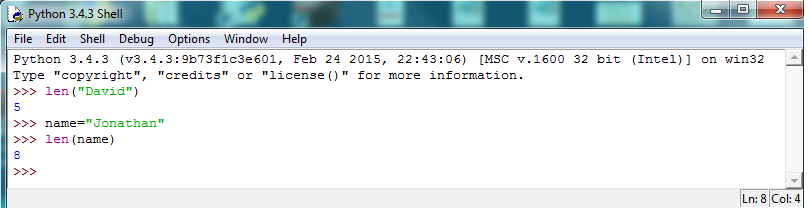﻿ How to Measure the Length of a String in Python ﻿# How to Measure the Length of a String in PythonIn this article, we show how to measure the length of a string in Python.

With Python, a string is measured through the len() function.

The len() function is a built-in PHP function that can measure any argument put it into it, whether a string or a number.

The function simply counts the number of characters.

The code to measure a string is shown below.

The line above measures the name, David, and it will return a value of 5.

The above code outputs the length of a string directly inserted to it.

To measure the length of a variable, simply enter in the variable into the len() function after it is initialized.

The code to do this is shown below.

This returns a value of 8, since Jonathan has 8 characters.

Lastly, we will sho how to get the length of a string from input.

Let's say we ask for his or her name.

The code to do so is shown below.

Now we get the length of the name that the user enters in.

So the len() function is the only thing required to get the length of a string in Python.

The output of this len() function is shown below.Related Resources

How to Open a New Web Browser Using Python